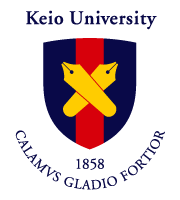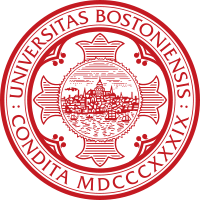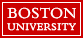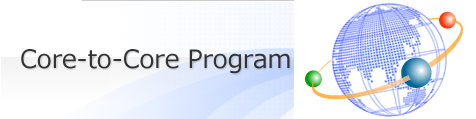BU-KEIO 2015 Schedule## Number Theory

### Boston University — September 8-12, 2015Schedule:

Abstracts for faculty talks:

• Ralph Greenberg (Univ. of Washington) -- Trivial zeroes

• The interpolation property defining p-adic L-functions sometimes implies the existence of a zero at a certain point. Several questions then emerge. First of all, what can you say about the first nonvanishing derivative at that point? Secondly, is there a corresponding behavior on the algebraic side which is consistent with the so-called "Main Conjectures" in Iwasawa theory? My talks will survey what is conjectured and what is known about these questions.

• Masato Kurihara (Keio Univ.) -- Iwasawa theory and p-adic L-functions

• I will discuss more precise relationship between several Iwasawa modules and p-adic L-functions than the usual main conjeture, using Fitting ideals, in the classical setting. This is joint work with C. Greither. I also talk on some related results on the relationship between arithmetic Galois modules and zeta values.

• Karl Rubin (Univ. of California-Irvine) -- Diophantine stability and Families of Selmer groups (two talks)

• Suppose V is an algebraic variety over a number field K, and V(K) is the set of K-rational points on V. For which fields L containing K is V(L) larger than V(K)? What does the collection of such extensions L/K tell us about V? In joint work with Barry Mazur we investigate these questions. For example, if V is an irreducible curve of genus at least one, then (possibly after increasing K) we produce a large collection of cyclic extensions L/K with V(L) = V(K).

• Selmer groups of elliptic curves and abelian varieties are the best way we know to get upper bounds for Mordell-Weil ranks. When two abelian varieties have isomorphic p-torsion subgroups (for example, when p = 2 and the two abelian varieties are quadratic twists of each other) we can try to compare the two p-Selmer groups. In joint work with Barry Mazur we study Selmer groups in families of twists, and give some applications.

• John Voight (Dartmouth) -- Presentations for rings of modular forms

• In these lectures, we show how to obtain explicit presentations for rings of modular forms. In the first lecture, we connect rings of modular forms to canonical rings, and we show how one obtains a complete description when the Fuchsian group acts freely; this extends the classical theorems of Max Noether and Petri to the case of a log curve. In the second lecture, we extend these results to a Fuchsian group of arbitrary signature; our main result is then an extension to the previous results to a general log stacky curve. This is joint work with David Zureick-Brown.

• Shuji Yamamoto (Keio Univ.) -- The Kaneko-Tsumura multiple zeta functions of r+r variables

• Recently, Kaneko and Tsumura introduced a new kind of multiple zeta functions with r complex variables and r non-positive integer parameters. In this talk, we study the extension of these functions to r+r complex variables and their values at positive integers.

Abstracts for contributed talks:

• John Bergdall (Boston Univ.) -- Slopes of modular forms and the ghost conjecture

• In this talk, we present a new conjecture on slopes of p-adic modular forms. We write down a relatively simple and explicit power series over weight space and conjecture, in the Gamma0(N)-regular case, that this power series knows the slopes of the Up operator acting on all spaces of overconvergent modular forms. Precisely, we conjecture that the Newton polygon of our series evaluated at a weight k (classical or not) matches the Newton polygon of the characteristic power series of Up acting on weight k overconvergent modular forms. We call this power series the "ghost series" as its spectral curve hovers around the true spectral curve. In this talk, we will explain this ghost conjecture and its connections to other conjectures on slopes (e.g. Buzzard's conjecture, the Gouvea-Mazur conjecture, Coleman's spectral halo) and discuss implications for the shape and structure of the eigencurve. This is a joint project with Rob Pollack.

• Michael Chou (Univ. of Connecticut) -- Torsion of Rational Elliptic Curves over Quartic Galois Number Fields

• The classification of the torsion subgroup of elliptic curves over Q was determined by Mazur. The classification over quadratic number fields was completed due to work of Kamienny, Kenku, and Momose. However, over cubic fields the classification is already incomplete. In this talk we discuss a refined version of this problem: let E be an elliptic curve defined over Q and K be a number field of degree d; what groups appear as E(K)_tor? In particular, we will present a classification over all quartic galois number fields K and show how the techniques used may be applied to other fields.

• Jessica Fintzen (Harvard Univ.) -- Stable vectors in the Moy-Prasad filtration

• Reeder and Yu gave recently a new construction of certain supercuspidal representations of p-adic reductive groups (called epipelagic representations). Their construction relies on the existence of stable vectors in the first Moy-Prasad filtration quotient under the action of a reductive quotient. We will explain these ingredients and present a theorem about the existence of such stable vectors for all primes p. This builds on a result of Reeder and Yu about the existence of stable vectors for large primes. The above work forms part of a joint research project with Beth Romano.

• Jorge Florez (CUNY) -- Explicit reciprocity laws for higher local fields

• In this talk we will describe a generalization of Kolyvagin's explicit reciprocity laws to higher dimensional local fields. Specifically, we will give formulas for the generalized Kummer pairing associated to a formal group in terms of multidimensional p-adic differentiation, the logarithm of the formal group and the generalized trace.

• Yoshinosuke Hirakawa (Keio Univ.) -- On the descent of certain modular Calabi-Yau varieties via the Cynk-Hulek construction

• In this talk, we introduce higher dimensional Calabi-Yau varieties which are certain 2-elementary quotients of products of K3 surfaces and give some arithmetic consequences.

• Yuichi Hirano (Tokyo Univ.) -- Congruences between Hilbert modular forms of weight 2 and the Iwasawa invariants.

• Let F be a totally real number field. In this talk, we present a way to show how congruences between a Hilbert cusp form over F and a Hilbert Eisenstein series over F of the same parallel weight 2 give rise to corresponding congruences between the special values of the associated (p-adic) L-functions. This is a generalization of the results of Vatsal in the case F=Q. Then we apply it to the Iwasawa main conjecture for a Hilbert cusp form of parallel weight 2 in the special case where the associated p-adic Galois representation is residually reducible. This is a partial generalization of the results of Greenberg and Vatsal in the case F=Q.

• Catherine Hsu (Univ. of Oregon) -- Two Classes of Number Fields with a Non-Principal Euclidean Ideal

• In 1979, Lenstra defined the Euclidean ideal, a generalization of the Euclidean algorithm. Just as the existence of a Euclidean algorithm for the ring of integers OK in a number field K implies a trivial class group, the existence of a Euclidean ideal C in OK implies a cyclic class group with generator [C]. By using certain growth results, Graves provided the first explicit example of a number field a non-principal Euclidean ideal. In this talk, we generalize Graves' techniques in order to introduce two classes of totally real quartic number fields, one of biquadratic extensions and one of cyclic extensions, each of which has a non-principal Euclidean ideal.

• Aditya Karnataki (Boston Univ.) -- On density of self-dual Artin representations

• Self-dual Artin representations should be rare to find. In particular, it is natural to expect that they occur with density 0. David Rohrlich proved results in this direction in dimensions 1 and 2. We present an extension of these results to dimension 3.

• Joseph Kramer-Miller (CUNY) -- p-adic L-functions on Hida families

• A major theme in the theory of p-adic deformations of automorphic forms is how p-adic L-functions over eigenvarieties relate to the geometry of these eigenvarieties. In this talk we explain results in this vein for the ordinary part of the eigencurve (i.e. Hida families). We address how Taylor expansions of one variable p-adic L-functions varying over families can detect geometric phenomena: crossing components of a certain intersection multiplicity and ramification over the weight space. Our methods involve proving a converse to a result of Vatsal relating congruences between eigenforms to their algebraic special L-values and then p-adically interpolating congruences using formal models. These methods should extend to the entire eigencurve.

• Jaclyn Lang (UCLA) -- Images of Galois representations associated to Hida families

• We explain a sense in which Galois representations associated to non-CM Hida families have large images. This is analogous to results of Ribet and Momose for Galois representations associated to classical modular forms. In particular, we show how extra twists of the Hida family decreases the size of the image.

• Anna Medvedovsky (ICERM) -- Lower bounds on dimensions of mod-p Hecke algebras

• In 2012, Nicolas and Serre revived interest in the study of Hecke algebras on modular forms mod p when they proved that for p = 2 the completed Hecke algebra is a power series ring over F_2 generated by T_3 and T_5. Their elementary arguments do not generalize directly to other primes, but their tools --- the Hecke recursion, the nilpotence filtration --- serve as the backbone of a new method-in-development, uniform and entirely in characteristic p, for understanding the structure of mod-p Hecke algebras. I will present this method (currently implemented for level one and p = 2, 3, 5, 7, 13) and compare it with Bellaiche-Khare, which uses characteristic-zero theory to prove similar results for all p>= 5.

• Tomoki Mihara (Tokyo Univ./Keio Univ.) -- A new geometric construction of a family of Galois representations associated to modular forms

• Let p be an odd prime number, and N >= 5 an integer divisible by p. We construct a pro-p Abelian sheaf on the modular curve of level Gamma_1(N) whose cohomology group contains all the Galois representations associated to a normalised cusidal eigenform of level Gamma_1(N) and weight >= 2. We cut the cohomology by a fixed bound of a slope, and verify its finiteness of the module over a certain universal Hecke algebra. For any family F of modular forms, the F-component of the Hecke module is a generically 2-dimensional Galois representation whose specialisations are the Galois representations associated to the modular forms given as the specialisations of F.

• Kazuaki Murakami (Keio Univ.) -- On the isomorphism classes of Iwasawa modules

• I will talk on the classification of finitely generated torsion Zp[[T]]-modules which are free over Zp of rank 3 and 4 with several examples of Iwasawa modules and Selmer modules.

• Hieu T. Ngo (Texas A & M - Qatar) -- Nonvanishing of Dirichlet L-functions in Galois orbits

• A classical theorem of Iwaniec and Sarnak states that at least one-third of the central values of Dirichlet L-functions do not vanish when the conductor approaches infinity. When the conductor is a power of a fixed prime, there are natural Galois actions that partition the set of primitive characters of that conductor into Galois orbits. We will discuss a strengthening of the Iwaniec-Sarnak theorem, showing that the one-third nonvanishing proportion holds within each Galois orbit. A key ingredient is the Ridout-Roth theorem, a deep result in Diophantine approximation. This is joint work with Rizwanur Khan and Djordje Milicevic

• Jiro Nomura (Keio Univ.) -- Annihilation of class groups for non-abelian CM-extensions

• Concerning the annihilation of class groups, the Brumer-Stark conjecture has been studied for many years. The conjecture is formulated for abelian extensions, however, over the last few years, a non-abelian generalization has been formulated independently by David Burns and Andreas Nickel. In this talk, I explain the formulation and known results. If time permits, I also introduce another non-abelian generalization due to G. Dejou and X.-F. Roblot. Finally, I explain my own results on these conjectures.

• Masataka Ono (Keio Univ.) -- Shuffle product of finite multiple polylogarithms

• In this talk, we introduce a finite sum analogue of multiple polylogarithms and a certain analogue of the shuffle product in an analogous framework of finite multiple zeta values. This is joint work with S. Yamamoto.

• Rei Otsuki (Keio Univ.) -- Nearly meromorphic periodic functions and CM elliptic curves

• In this talk, we will define a kind of generalization of elliptic functions, which we call a nearly meromorphic periodic function. We will discuss algebraicity and p-adic divisibility of the special values of such functions when the period lattice corresponds to a CM elliptic curve. As an application, we will introduce a construction of p-adic distribution using the Eisenstein-Kronecker functions.

• Bharathwaj Palvannan (Univ. of Washington) -- Algebraic analog of p-adic factorization

• The main conjecture of Iwasawa theory predicts a close relationship between Selmer groups and p-adic L-functions. Our work is motivated by the following results on p-adic L-functions. 1) Dasgupta's factorization of Hida's 3 variable p-adic L-function as a product of a Coates-Schmidt 2-variable p-adic L-function (associated to Sym^2) and a Kubota-Leopoldt p-adic L-function. 2) Gross's factorization of Katz's 2-variable p-adic L-function as a product of two Kubota-Leopoldt p-adic L-functions. We develop the corresponding results involving Selmer groups that are predicted by the main conjecture (Our results involving Selmer groups do not rely on the validity of the main conjecture). Another example that fits into this framework involves Hida families whose residual Galois representation is reducible. This example involves the application of Ohta's work on the Eisenstein ideal. "

• Takamichi Sano (Keio Univ.) -- On the arithmetic of generalized Stark elements

• I will give a generalization of Stark elements for twisted Tate motives. I will discuss some arithmetic properties of the generalized Stark elements, and their relations with the equivariant Tamagawa number conjecture. This is joint work with D. Burns and M. Kurihara.

• Florian Sprung (Princeton Univ.) -- What makes the rank of an elliptic curve grow in cyclotomic towers?

• For an elliptic curve E/Q and a prime p of good reduction, the Mordell-Weil rank remains bounded in the cyclotomic Z_p-extension via a result of Kato (algebraic side) / Rohrlich (analytic side). Some natural questions to ask are: 1. What is this bound? 2. Where does it come from? In the ordinary case, the lambda-invariant associated to the Selmer group is an upper bound. A natural question to ask is what happens in the supersingular case. Here, Kobayashi and Pollack each gave algebraic resp. analytic bounds when a_p=0. We first present a different analytic bound involving Kurihara terms that works for the general supersingular case. This is also an algebraic bound in view of the Iwasawa main conjecture, but this line of proof doesn't answer question 2. We present an idea that explains the appearance of the Kurihara terms, in the case a_p=0.

• Yuuki Takai (Keio Univ.) -- On non-vanishing modulo p of central values of L-functions over totally real fields.

• This talk is about some mod p non-vanishing results of quadratic twists of L-functions over totally real fields by using Hilbert modular forms of half-integral weights. In particular, I will mainly show indivisibility of relative class numbers of CM quadratic extension of a fixed totally real field. If time permits, I will also explain an attempt to show a similar result for Hilbert modular L-functions.

• Carl Wang-Erickson (Brandeis Univ.) -- The order of vanishing of a Kubota-Leopoldt zeta-function

• Fix a point in an ordinary eigencurve where a cuspidal family intersects the Eisenstein family. At this point, the Kubota-Leopoldt p-adic zeta-function giving the constant term of the Eisenstein series vanishes. Joint work with Preston Wake (UCLA) has established an isomorphism between the complete local ring at that point and an ordinary Galois deformation ring, subject to assumptions on class groups. The zeta-function influences the structure of this local ring, while Galois cohomology controls the structure of the Galois deformation ring. I will discuss how certain "higher cup products" in Galois cohomology influence the structure of the Galois deformation ring, which in turn controls the order of vanishing of the zeta-function. This extends work of Fukaya and Kato, which addresses vanishing of order 2 and the classical cup product.

• Sandi Xhumari (Univ. of Connecticut) -- The Gross-Koblitz formula and generalized Gauss sums

• The Gross-Koblitz formula expresses Gauss sums over finite fields essentially as a product of values of the p-adic Gamma function at rational numbers, and it is a p-adic lifting of Stickelberger's congruence for Gauss sums. In this talk I will outline a proof of the Gross-Koblitz formula by Dwork and Lang. It relies on a differential operator whose index on formal power series and overconvergent p-adic power series are not the same. I will then discuss how these ideas extend to generalized Gauss sums.

• Kazuki Yamada (Keio Univ.) -- Log rigid syntomic cohomology for strictly semistable schemes

• In this talk, we will construct the log rigid syntomic cohomology for strictly semistable schemes by using Grosse-Klonne's log rigid cohomology. It is a p-adic analogue of the absolute Hodge cohomology.

• Myungjun Yu (Univ. of California-Irvine) -- Selmer ranks of Jacobians of hyperelliptic curves in families of quadratic twists

• Mazur and Rubin found sufficient conditions for elliptic curves to have infinitely many quadratic twists that have 2 Selmer ranks r, for any given non-negative integer r. We generalize this result to hyperelliptic curves.